Share filter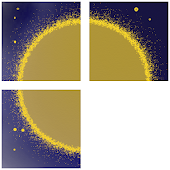Developed by in Education category

4/5.0 Ratings

1,000 - 5,000 Installs

12 Reviews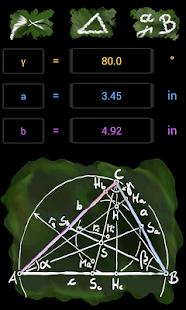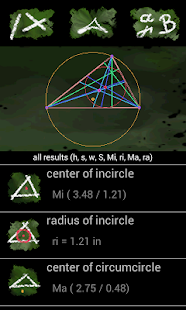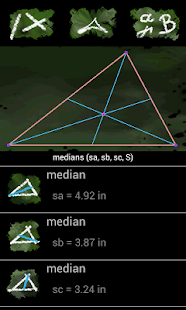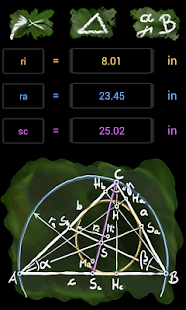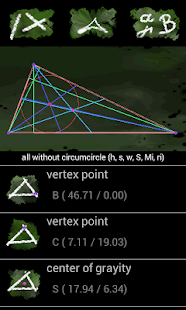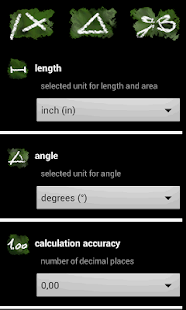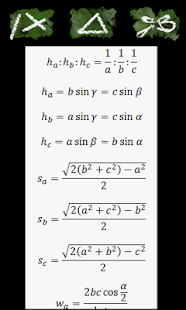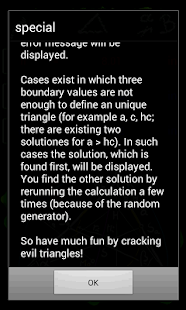### Description

The free version Calc Triangle Solver is one of the best and high performanced triangle cracker on the marked. This section of geometry calculates and solves all possible triangles: equilateral triangle, right-angled triangle and oblique-angled triangle!

With any three input parameter you like (length of side, angle, bisector of angle, median, height, incircle, circumcircle,...) the Calc Triangle Solver calculates all geometric relevant results (inclusive center of gravity, center of incircle, center of circumcirle, ...).

The triangle is exactly geometrically drawn. Additionally the heights, the medians, the bisectors, the incircle and the circumcircle can be displayed.

Optionally you are able to choose between the metric-system (mm, cm, m) and the inch-system (inch, foot, yard). The angles are evaluated optionally in degrees, radians or gradians.

A petite formulary lists important triangle theorems like the sine theorem, the cosine theorem, ...
If the input is incorrect, the Calc Triangle Solver searches for an alternative proposal.

This app is useful for beginners and experts.

Hint for experts: In a few of cases there exists no solution for some boundary conditions (for example ri > ra or a > b + c). In this cases no result will be shown and an error message will be displayed. Cases exist in which three boundary values are not enough to define an unique triangle (for example a, c, hc; there are existing two solutions for a > hc). In such cases it will be displayed the solution, which is found at first. You find the other solutions by rerunning the calculation a few times (because of the random generator.

So have much fun by cracking evil triangles!

This content has been crawled through Play Store, we don't store any APK file.